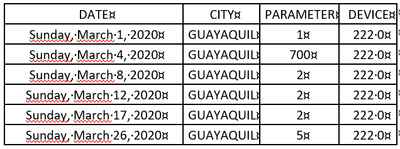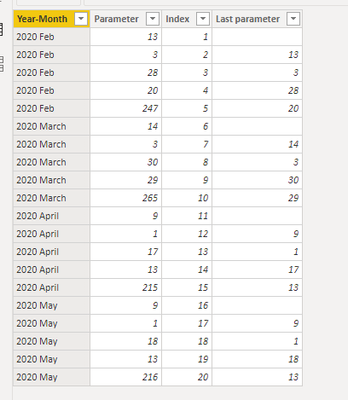cancel
Showing results for
Did you mean:Helper III

## How to create a column or measure for to search the last value of a determinate column

Hi everyone, everything fine? I need help, I have a problem and I don't know what to do. I have the next table:I need create a column or measure that showing the last value of PARAMETER from device on the month. The idea is to create a bar graph with the amount of devices that went send a determinate value:In the graph each bar is a parameter value, this bar graph is showing the number of devices that sent each parameter value, I need these parameter values to be the last ones sent in each month.

Can someone help me make this column or measure? Thank you all for the attention!

1 ACCEPTED SOLUTIONCommunity Support

Hi  @diegolima ,

Is below what you need?If so,first go to query editor>add column>index column;

Then create a calculated column as below:

``Last parameter = CALCULATE(MAX('Table'[Parameter]),FILTER('Table','Table'[Index]=EARLIER('Table'[Index])-1&&'Table'[Year-Month]=EARLIER('Table'[Year-Month])))``

Best Regards,
Kelly
5 REPLIES 5Community Support

Hi  @diegolima ,

Is below what you need?If so,first go to query editor>add column>index column;

Then create a calculated column as below:

``Last parameter = CALCULATE(MAX('Table'[Parameter]),FILTER('Table','Table'[Index]=EARLIER('Table'[Index])-1&&'Table'[Year-Month]=EARLIER('Table'[Year-Month])))``

Best Regards,
KellyHelper III

Thanks for answer, but I don't understand one thing, the column Index is equal the column device of the my table? If yes what the value to be showing with last value?Community Support

Hi @diegolima ,

Index column is similar to rank your rows ascending.So  the last value equals to the value of the row whose index refers to the current row index -1.

Is my result what you need?

Best Regards,
KellySuper User

Seems like you want LOOKUPVALUE or Lookup Min/Max here: https://community.powerbi.com/t5/Quick-Measures-Gallery/Lookup-Min-Max/m-p/985814#M434

Become an expert!: Enterprise DNA
External Tools: MSHGQM
Latest book!:
Mastering Power BI 2nd EditionDAX is easy, CALCULATE makes DAX hard...Helper III

Thanks Greg for the answer, but I still don't understand how to solve my problem, I saw in the other post that you sent a question similar to my problem. I didn't see how to generate a column or measure to highlight the last parameter values ​​in the month.

Thank you so much!